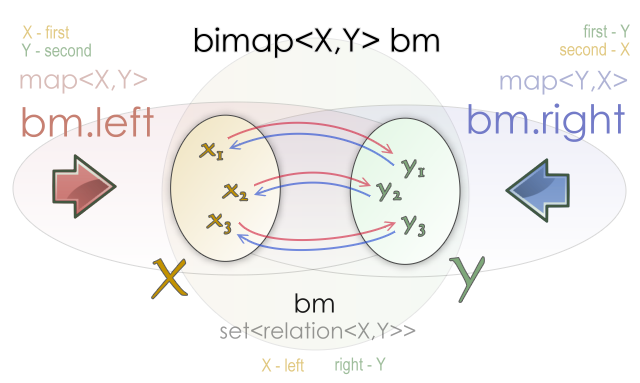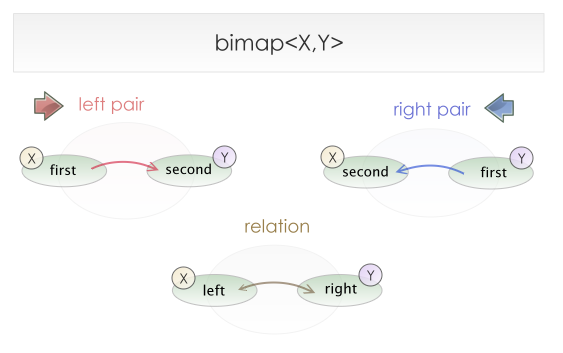#Boost C++ Libraries

...one of the most highly regarded and expertly designed C++ library projects in the world.

This is the documentation for an old version of Boost. Click here to view this page for the latest version.

## One minute tutorial

#### What is a bimap?

A Bimap is a data structure that represents bidirectional relations between elements of two collections. The container is designed to work as two opposed STL maps. A bimap between a collection `X` and a collection `Y` can be viewed as a map from `X` to `Y` (this view will be called the left map view) or as a map from `Y` to `X` (known as the right map view). Additionally, the bimap can also be viewed as a set of relations between `X` and `Y` (named the collection of relations view).

The following code creates an empty bimap container:

```typedef bimap<X,Y> bm_type;
bm_type bm;
```

Given this code, the following is the complete description of the resulting bimap. 

• `bm.left` is signature-compatible with `std::map<X,Y>`
• `bm.right` is signature-compatible with `std::map<Y,X>`
• `bm` is signature-compatible with `std::set< relation<X,Y> >`You can see how a bimap container offers three views over the same collection of bidirectional relations.

If we have any generic function that work with maps

```template< class MapType >
void print_map(const MapType & m)
{
typedef typename MapType::const_iterator const_iterator;
for( const_iterator iter = m.begin(), iend = m.end(); iter != iend; ++iter )
{
std::cout << iter->first << "-->" << iter->second << std::endl;
}
}
```

We can use the left map view and the right map view with it

```bimap< int, std::string > bm;
...
print_map( bm.left  );
print_map( bm.right );
```

And the output will be

````1 --> one`
`2 --> two`
...
`one --> 1`
`two --> 2`
...
```

#### Layout of the relation and the pairs of a bimap

The `relation` class represents two related elements. The two values are named left and right to express the symmetry of this type. The bimap pair classes are signature-compatible with `std::pairs`.#### Step by step

A convenience header is available in the boost directory:

```#include <boost/bimap.hpp>
```

Lets define a bidirectional map between integers and strings:

```typedef boost::bimap< int, std::string > bm_type;
bm_type bm;
```

#### The collection of relations view

Remember that `bm` alone can be used as a set of relations. We can insert elements or iterate over them using this view.

```bm.insert( bm_type::value_type(1, "one" ) );
bm.insert( bm_type::value_type(2, "two" ) );

std::cout << "There are " << bm.size() << "relations" << std::endl;

for( bm_type::const_iterator iter = bm.begin(), iend = bm.end();
iter != iend; ++iter )
{
// iter->left  : data : int
// iter->right : data : std::string

std::cout << iter->left << " <--> " << iter->right << std::endl;
}
```

#### The left map view

`bm.left` works like a ```std::map< int, std::string >```. We use it in the same way we will use a standard map.

```typedef bm_type::left_map::const_iterator left_const_iterator;

for( left_const_iterator left_iter = bm.left.begin(), iend = bm.left.end();
left_iter != iend; ++left_iter )
{
// left_iter->first  : key  : int
// left_iter->second : data : std::string

std::cout << left_iter->first << " --> " << left_iter->second << std::endl;
}bm_type::left_const_iterator left_iter = bm.left.find(2);
assert( left_iter->second ==  "two" );bm.left.insert( bm_type::left_value_type( 3, "three" ) );
```

 The type of `bm.left` is `bm_type::left_map` and the type of `bm.right` is `bm_type::right_map` `bm_type::left_`-type- can be used as a shortcut for the more verbose `bm_type::left_map::`-type- This line produces the same effect of ```bm.insert( bm_type::value_type(3,"three") );```

#### The right map view

`bm.right` works like a ```std::map< std::string, int >```. It is important to note that the key is the first type and the data is the second one, exactly as with standard maps.

```bm_type::right_const_iterator right_iter = bm.right.find("two");

// right_iter->first  : key  : std::string
// right_iter->second : data : int

assert( right_iter->second ==  2 );

assert( bm.right.at("one") == 1 );

bm.right.erase("two");bm.right.insert( bm_type::right_value_type( "four", 4 ) );
```

 This line produces the same effect of ```bm.insert( bm_type::value_type(4,"four") );```

#### Differences with std::map

The main difference between bimap views and their standard containers counterparts is that, because of the bidirectional nature of a bimap, the values stored in it can not be modified directly using iterators. For example, when a `std::map<X,Y>` iterator is dereferenced the return type is ```std::pair<const X, Y>```, so the following code is valid: `m.begin()->second = new_value;`. However dereferencing a `bimap<X,Y>::left_iterator` returns a type that is signature-compatible with a `std::pair<const X, const Y>`

```bm.left.find(1)->second = "1"; // Compilation error
```

If you insert `(1,"one")` and `(1,"1")` in a `std::map<int,std::string>` the second insertion will have no effect. In a `bimap<X,Y>` both keys have to remain unique. The insertion may fail in other situations too. Lets see an example

```bm.clear();

bm.insert( bm_type::value_type( 1, "one" ) );

bm.insert( bm_type::value_type( 1, "1"   ) ); // No effect!
bm.insert( bm_type::value_type( 2, "one" ) ); // No effect!

assert( bm.size() == 1 );
```

#### A simple example

Look how you can reuse code that is intend to be used with std::maps, like the print_map function in this example.

```#include <string>
#include <iostream>

#include <boost/bimap.hpp>

template< class MapType >
void print_map(const MapType & map,
const std::string & separator,
std::ostream & os )
{
typedef typename MapType::const_iterator const_iterator;

for( const_iterator i = map.begin(), iend = map.end(); i != iend; ++i )
{
os << i->first << separator << i->second << std::endl;
}
}

int main()
{
// Soccer World cup

typedef boost::bimap< std::string, int > results_bimap;
typedef results_bimap::value_type position;

results_bimap results;
results.insert( position("Argentina"    ,1) );
results.insert( position("Spain"        ,2) );
results.insert( position("Germany"      ,3) );
results.insert( position("France"       ,4) );

std::cout << "The number of countries is " << results.size()
<< std::endl;

std::cout << "The winner is " << results.right.at(1)
<< std::endl
<< std::endl;

std::cout << "Countries names ordered by their final position:"
<< std::endl;

// results.right works like a std::map< int, std::string >

print_map( results.right, ") ", std::cout );

std::cout << std::endl
<< "Countries names ordered alphabetically along with"
"their final position:"
<< std::endl;

// results.left works like a std::map< std::string, int >

print_map( results.left, " ends in position ", std::cout );

return 0;
}
```

The output of this program will be the following:

````The number of countries is 4`

`The winner is Argentina`

`Countries names ordered by their final position:`
`1) Argentina`
`2) Spain`
`3) Germany`
`4) France`

`Countries names ordered alphabetically along with their final position:`
`Argentina ends in position 1`
`France ends in position 4`
`Germany ends in position 3`
`Spain ends in position 2`
```

#### Continuing the journey

For information on function signatures, see any standard library documentation or read the reference section of this documentation.

CautionBe aware that a bidirectional map is only signature-compatible with standard containers. Some functions may give different results, such as in the case of inserting a pair into the left map where the second value conflicts with a stored relation in the container. The functions may be slower in a bimap because of the duplicated constraints. It is strongly recommended that you read The full tutorial if you intend to use a bimap in a serious project.

 A type is signature-compatible with other type if it has the same signature for functions and metadata. Preconditions, postconditions and the order of operations need not be the same.

 Copyright © 2006-2012 Matias Capeletto Distributed under the Boost Software License, Version 1.0. (See accompanying file LICENSE_1_0.txt or copy at http://www.boost.org/LICENSE_1_0.txt)